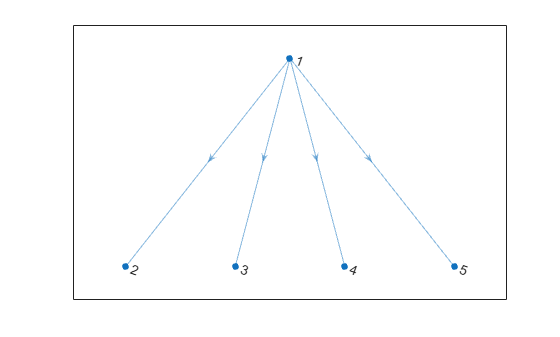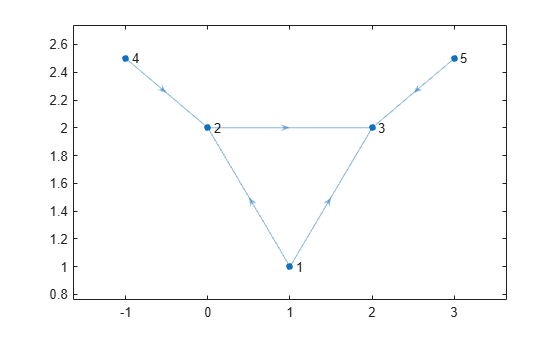flipedge

Reverse edge directions

Description

example

H = flipedge(G) returns a directed graph that has the same edges as G, but with reversed directions. H contains the same node and edge properties as G.

example

H = flipedge(G,s,t) reverses a subset of edges using the node pairs s and t.

H = flipedge(G,idx) reverses a subset of edges using the edge indices idx.

Examples

collapse all

Create and plot a directed graph. Then reverse the direction of all of the edges in the graph.

G = digraph([1 1 1 1],[2 3 4 5]);
plot(G)H = flipedge(G);
plot(H)Create and plot a directed graph. Specify custom xy node coordinates for the plot.

G = digraph([1 1 2 2 3],[2 3 3 4 5]);
x = [1 0 2 -1 3];
y = [1 2 2 2.5 2.5];
plot(G,'XData',x,'YData',y)Reverse the direction of the edges (2,4) and (3,5), and then replot the graph.

H = flipedge(G,[2 3],[4 5]);
plot(H,'XData',x,'YData',y)Input Arguments

collapse all

Input graph, specified as a digraph object. Use digraph to create a directed graph object.

Example: G = digraph([1 2],[2 3])

Node pairs, specified as separate arguments of node indices or node names. Similarly located elements in s and t specify the source and target nodes for edges in the graph.

This table shows the different ways to refer to one or more nodes either by their numeric node indices or by their node names.

FormSingle NodeMultiple Nodes
Node index

Scalar

Example: 1

Vector

Example: [1 2 3]

Node name

Character vector

Example: 'A'

Cell array of character vectors

Example: {'A' 'B' 'C'}

String scalar

Example: "A"

String array

Example: ["A" "B" "C"]

Example: H = flipedge(G,[1 4],[2 3]) reverses the direction of the edges (1,2) and (4,3).

Example: H = flipedge(G,{'a' 'd'},{'b' 'c'}) reverses the direction of the edges (a,b) and (d,c).

Edge indices, specified as a scalar or vector of positive integers. Each edge index corresponds to a row in the G.Edges table of the graph G.Edges(idx,:).

Example: H = flipedge(G,3) flips the direction of edge G.Edges(3,:).

Output Arguments

collapse all

Output graph, returned as a digraph object. Compared to G, H has the same nodes but some or all of the edges are in reversed direction. H also has the same node and edge properties as G.

Introduced in R2016b